Related Articles

# Time series data Visualization in Python

• Last Updated : 15 Mar, 2021

A time series is the series of data points listed in time order. A time series is a sequence of successive equal interval points in time. A time-series analysis consists of methods for analyzing time series data in order to extract meaningful insights and other useful characteristics of data. Time-series data analysis is becoming very important in so many industries like financial industries, pharmaceuticals, social media companies, web service providers, research, and many more. To understand the time-series data, visualizations are essential. Any type of data analysis is not complete without visualizations. Because one good visualization can provide meaningful and interesting insights into data.

To do any type of data analysis dataset is the most important and basic requirement. Without a dataset, we can not perform analysis. Here we are taking stock data for time series data visualization. Click here to view the complete Dataset. For Visualizing time series data we need to import some packages:

## Python3

 `import` `pandas as pd``import` `numpy as np``import` `matplotlib.pyplot as plt`

## Python3

 `# reading the dataset using read_csv``df ``=` `pd.read_csv(``"stock_data.csv"``, ``                 ``parse_dates``=``True``, ``                 ``index_col``=``"Date"``)`` ` `# displaying the first five rows of dataset``df.head()`

Output: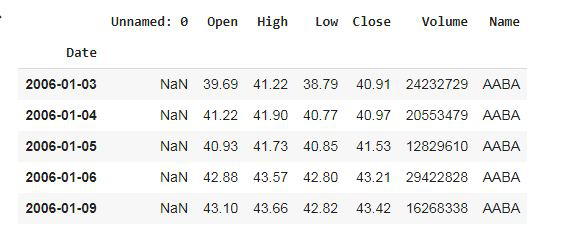We have used the ‘parse_dates’ parameter in the read_csv function to convert the ‘Date’ column to the DatetimeIndex format. By default, Dates are stored in string format which is not the right format for time series data analysis.

Now, removing the unwanted columns from dataframe i.e. ‘Unnamed: 0’.

## Python3

 `# deleting column``df.drop(columns``=``'Unnamed: 0'``)`

Output: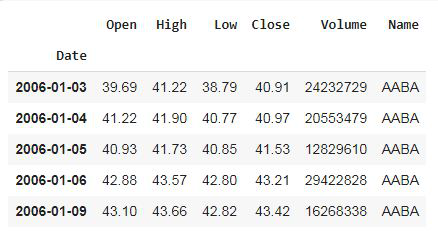Example 1: Plotting a simple line plot for time series data.

## Python3

 `df[``'Volume'``].plot()`

Output: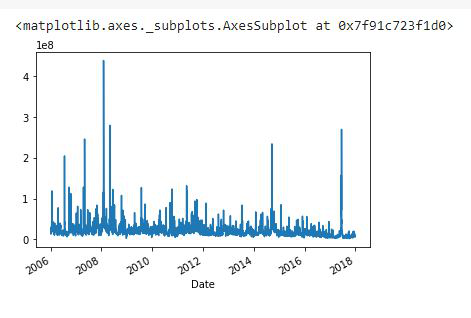Here, we have plotted the ‘Volume’ column data.

Example 2: Now let’s plot all other columns using subplot.

## Python3

 `df.plot(subplots``=``True``, figsize``=``(``10``, ``12``))`

Output: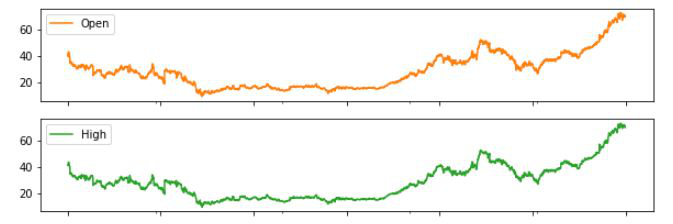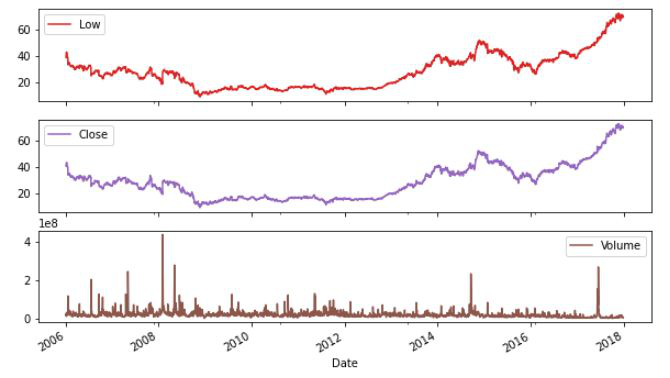The line plots used above are good for showing seasonality.

Seasonality: In time-series data, seasonality is the presence of variations that occur at specific regular time intervals less than a year, such as weekly, monthly, or quarterly.

Resampling: Resampling is a methodology of economically using a data sample to improve the accuracy and quantify the uncertainty of a population parameter. Resampling for months or weeks and making bar plots is another very simple and widely used method of finding seasonality. Here we are going to make a bar plot of month data for 2016 and 2017.

Example 3:

## Python3

 `# Resampling the time series data based on monthly 'M' frequency``df_month ``=` `df.resample(``"M"``).mean()`` ` `# using subplot``fig, ax ``=` `plt.subplots(figsize``=``(``10``, ``6``))`` ` `# plotting bar graph``ax.bar(df_month[``'2016'``:].index, ``       ``df_month.loc[``'2016'``:, ``"Volume"``], ``       ``width``=``25``, align``=``'center'``)`

Output: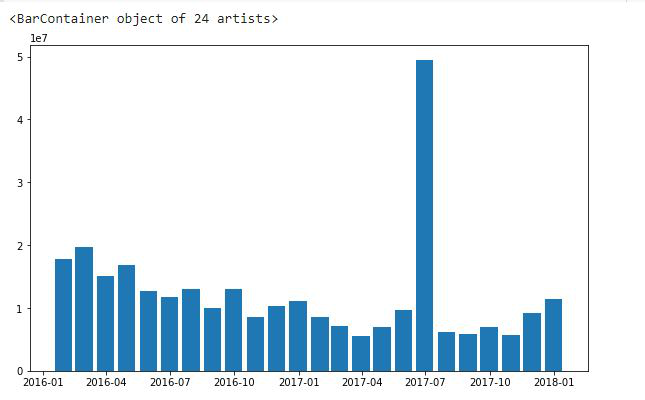There are 24 bars in the graph and each bar represents a month.

Differencing: Differencing is used to make the difference in values of a specified interval. By default, it’s one, we can specify different values for plots. It is the most popular method to remove trends in the data.

## Python3

 `df.Low.diff(``2``).plot(figsize``=``(``10``, ``6``))`

Output: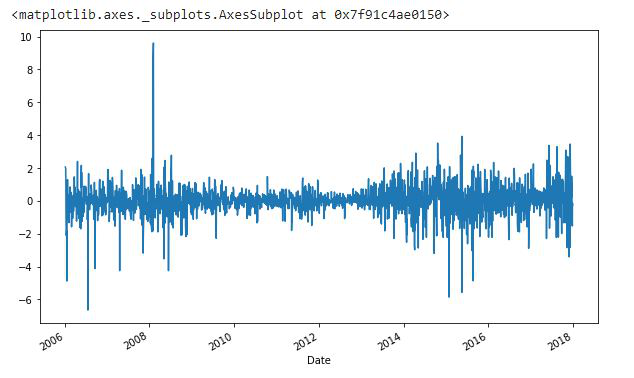## Python3

 `df.High.diff(``2``).plot(figsize``=``(``10``, ``6``))`

Output: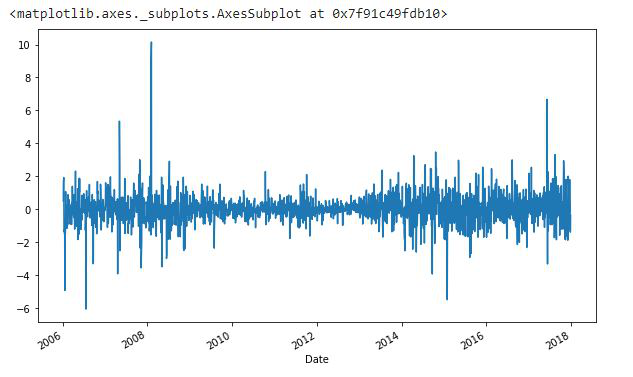### Plotting the Changes in Data

We can also plot the changes that occurred in data over time. There are a few ways to plot changes in data.

Shift: The shift function can be used to shift the data before or after the specified time interval. We can specify the time, and it will shift the data by one day by default. That means we will get the previous day’s data. It is helpful to see previous day data and today’s data simultaneously side by side.

## Python3

 `df[``'Change'``] ``=` `df.Close.div(df.Close.shift())``df[``'Change'``].plot(figsize``=``(``10``, ``8``), fontsize``=``16``)`

In this code, .div() function helps to fill up the missing data values. Actually, div() means division. If we take df. div(6) it will divide each element in df by 6. We do this to avoid the null or missing values that are created by the ‘shift()’ operation.

Here, we have taken .div(df.Close.shift()), it will divide each value of df to df.Close.shift() to remove null values.

Output: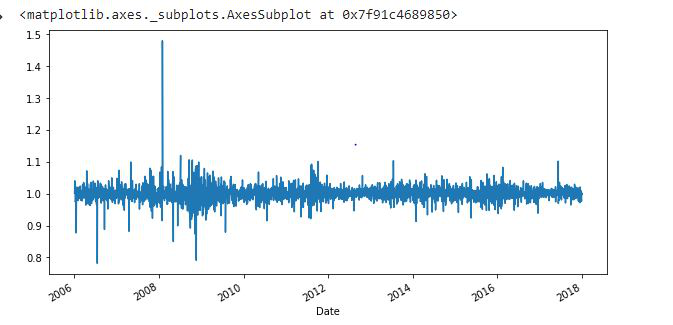We can also take a specific interval of time and plot to have a clearer look. Here we are plotting the data of only 2017.

## Python3

 `df[``'2017'``][``'Change'``].plot(figsize``=``(``10``, ``6``))`

Output: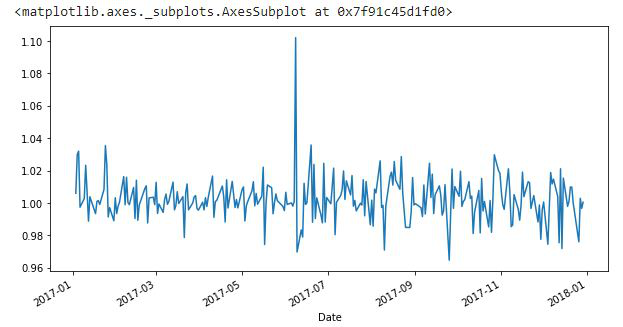Attention geek! Strengthen your foundations with the Python Programming Foundation Course and learn the basics.

To begin with, your interview preparations Enhance your Data Structures concepts with the Python DS Course. And to begin with your Machine Learning Journey, join the Machine Learning – Basic Level Course

My Personal Notes arrow_drop_up